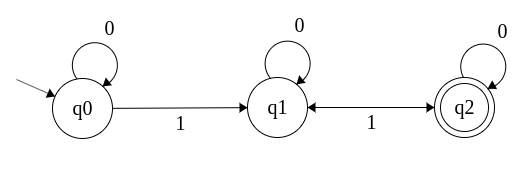Not Providing Milkshakes

# npm

Miss any of our Open RFC calls?Watch the recordings here! »

## dfa-machine

1.0.2 • Public • Published

# dfa-machine

A simple implementation of a dynamic finite state machine represinting an instance of a DFA (deterministic finite automata)

# Installation

Install `dfa-machine` using npm with the command `\$ npm install dfa-machine --save`

# Abstract

Any deterministic finite automata is defined by five tuples (Q,Σ,q0,δ,F) where:

• Q: The set of all states
• Σ (sigma): The set of all accepted inputs
• q0: The initial or starting state
• δ (delta): The transition function `δ: Q X Σ ⟶ Q`
• F: The set of final/accepted states

# Illustration

`dfa-machine` uses the same concept and implements it in javascript as follows: The constructor of the DFA accpets two arguments:

1. sigma: an array of all accepted inputs The current version supports inputs of the types `String`, `Number`, and `Boolean` only
2. machine object: an object describing initial state, final state(s), all states, and the trnsition functions/course for each state.
• The key `initial` must be present and have the value of a string represinting the name/key of the initial state which will be provided in the states object.
• The key `final` must be present and have the value of an array describing the states which will be considered accepted as a final states for a given string to be validated.
• The key `states` must be present and have the value of an object describing each state as a key (including but not limited to the initial and final states).
• `states` key must have the value of an object such as `{on: {input1: 'next-state', input2: 'next-state', ...etc}}` which will describe the transition function for each state while reading all inputs to be applied.

# Usage

In this example we will create a dfa on `Σ = {0,1}` that accepts strings of length greater than 0 with an even number of `1`s which described in the below figure.Our states:

• q0: The initial state. It represents either an empty string or a string with no `1`s in it (non-final).
• q1: Represents an odd number of `1`s (non-final).
• q2: Represents an even number of `1`s (final).

With that in mind we will setup our `DFA` instance as follows:

# Examples

See more examples here: https://github.com/ahmedisam99/dfa-machine/tree/master/examples

MIT

## Keywords

### Install

`npm i dfa-machine`

5

1.0.2

MIT

25.8 kB

12